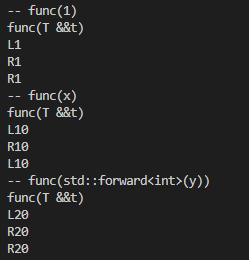> 文档中心 > c++左值、右值引用和移动语义

# c++左值、右值引用和移动语义

### c++左值、右值引用和移动语义

• 一、左值和右值
• 二、左值引用&
• 三、右值引用&&
• 应用：右值引用优化性能，避免深拷贝
• 四、移动语义 move
• 五、完美转发forward

C++11中引用了右值引用和移动语义，可以避免无谓的复制，提高了程序性能。

# 一、左值和右值

``int a=10;int b=a;``

# 二、左值引用&

`&`只能用作左值引用，因此对于下面的`test(int& x)`，中的参数x一定是左值

``void test(int& x){//这个x一定是左值    cout<<x<<endl;}int main(){    int a=10;    //test(10); //传入的是右值，因此会报错    test(a); //传入的是左值}``

# 三、右值引用&&

``void test(int&& x){    cout<<x<<endl;}int main(){    int a=10;    test(10); }``

&&总结：

• 左值和右值是独立于它们的类型的，右值引用类型可能是左值也可能是右值（也就是说对于&，只能用作左值引用，而&&既可以作为左值引用也可以作为右值引用，取决于传入的参数是左值还是右值）
• auto&& 或函数参数类型自动推导的 T&& 是一个未定的引用类型，被称为 universal references，
它可能是左值引用也可能是右值引用类型，取决于初始化的值类型。
• 所有的右值引用叠加到右值引用上仍然是一个右值引用，其他引用折叠都为左值引 用。当 T&& 为
模板参数时，输入左值，它会变成左值引用，而输入右值时则变为具名的右 值引用
• 编译器会将已命名的右值引用视为左值，而将未命名的右值引用视为右值

## 应用：右值引用优化性能，避免深拷贝

`A(const A& a)`是拷贝构造函数，如果没有移动构造函数`A(A&& a)`的情况下，执行 `A a = Get(false)`，调用的是拷贝构造函数，它会将Get(flase)返回的结果拷贝给a，然后执行析构函数，但是这样会造成性能的损耗。

（创建和销毁）对性能的影响。

``#include using namespace std;class A{public:    A() :m_ptr(new int(0)) { cout << "constructor A"  << endl;    }    A(const A& a) :m_ptr(new int(*a.m_ptr)) { cout << "copy constructor A"  << endl;    }    A(A&& a) :m_ptr(a.m_ptr) { a.m_ptr = nullptr; cout << "move  constructor A"  << endl;    }    ~A(){ cout << "destructor A, m_ptr:" << m_ptr  << endl; if(m_ptr)     delete m_ptr;    }private:    int* m_ptr;};// 为了避免返回值优化，此函数故意这样写A Get(bool flag){    A a;    A b;    cout << "ready return" << endl;    if (flag) return a;    else return b;}int main(){    { A a = Get(false); // 正确运行    }    cout << "main finish" << endl;    return 0;}``

# 四、移动语义 move

``int main(){MyString a;a = MyString("Hello"); // moveMyString b = a; // copyMyString c = std::move(a); // move， 将左值转为右值return 0;}``

# 五、完美转发forward

forward 完美转发实现了参数在传递过程中保持其值属性的功能，即若是左值，则传递之后仍然是左

``Template<class T>void func(T &&val);``

``int &&a = 10;int &&b = a; //错误``

``int &&a = 10;int &&b = std::forward<int>(a);``

``#include using namespace std;template <class T>void Print(T &t){    cout << "L" << t << endl;}template <class T>void Print(T &&t){    cout << "R" << t << endl;}template <class T>void func(T &&t) // 左值右值都只能走这个{    cout << "func(T &&t)" << endl;    Print(t);  // L   (什么都不做，默认当作左值)    Print(std::move(t));// 肯定调用"R"    Print(std::forward<T>(t)); // 不确定L  R  ,如果要完美往下层函数转发加forward}int main(){    cout << "-- func(1)" << endl;    func(1);  //  1是R    int x = 10;    int y = 20;    cout << "-- func(x)" << endl;    func(x);  // x本身是左值     （因此后续调用Print(std::forward(t))的时候，传入的依然是左值）    cout << "-- func(std::forward(y))" << endl;    func(std::forward<int>(y)); // std::forward(y)变成右值  （如果传入的参数，本身不带有&&符号，那么forward就把它当作右值）    return 0;}``